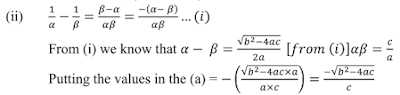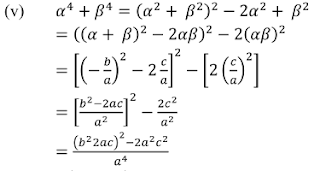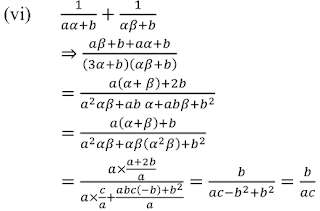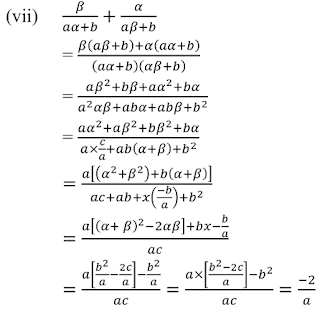## Chapter 2 Polynomials R.D. Sharma Solutions for Class 10th Math Exercise 2.1

Exercise 2.1

1. Find the zeroes of each of the following quadratic polynomials and verify the relationship between the zeroes and their co efficient:
(i) f(x) = x2 - 2x - 8
(ii) g(s) = 4s2 - 4x + 1
(iii) h(t) = t2 - 15
(iv) p(x) = x2 +2√2x + 6
(v) q(x) = √3x2 + 10x + 7√3
(vi) f(x) = x2 - (√3+1)x + √3
(vii) g(x) = a(x2 +1) - x(a2 +1)
(viii) 6x2 - 3 - 7x

Solution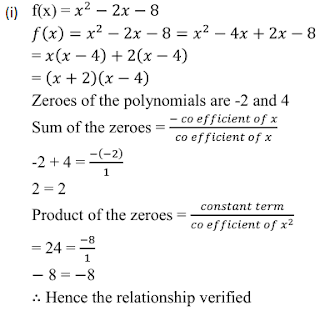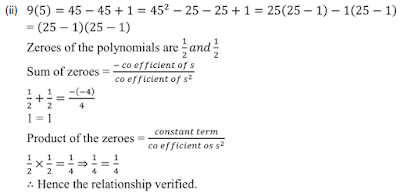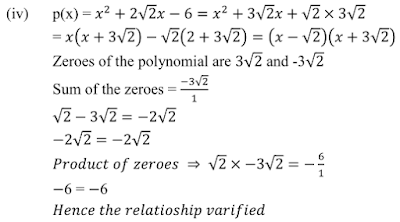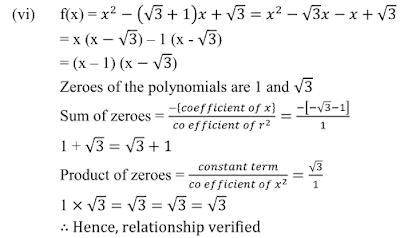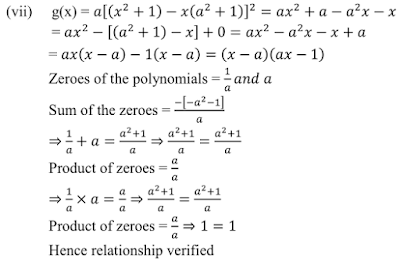2. For each of the following , find a quadratic polynomial whose sum and product respectively of the zeroes are as give . Also, find the zeroes of these polynomials by factorization.
(i) -8/3, 4/3
(ii) 21/8, 5/16
(iii) -2√3, -9
(iv) -3/2√5, -1/2

Solution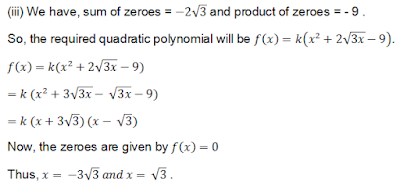3. If α and β are the zeros of the quadratic polynomial f(x) = x2 – 5x + 4, find the value of 1/α + 1/ β - 2 αβ.

Solution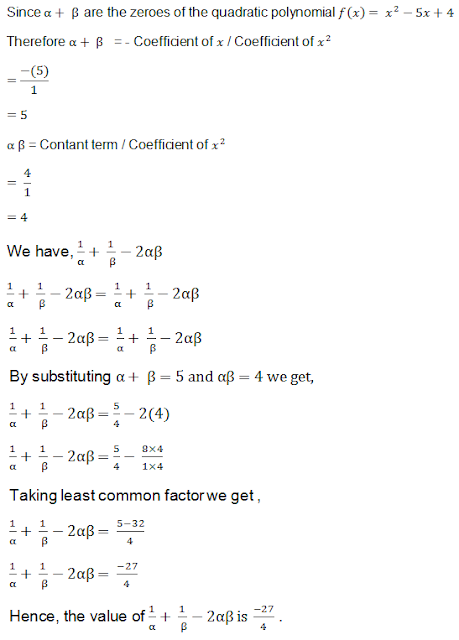4. If α and β are the zeros of the quadratic polynomial p(y) = 5y2 - 7y + 1, find the value of 1/α + 1/β - 2αβ .

Solution5. If α and β are the zeros of the quadratic polynomial f(x) = x2 - x - 4, find the value of 1/α + 1/ β - αβ .

Solution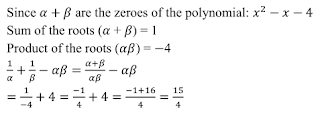6. If α and β are the zeros of the quadratic polynomial f(x) = x2 + x - 2, find the value of 1/α - 1/ β .

Solution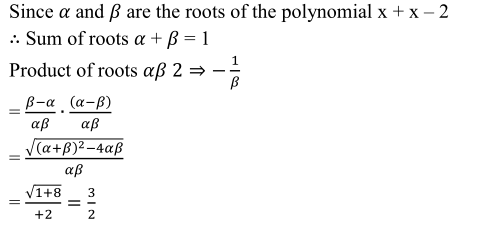7. If one zeros of the quadratic polynomial f(x) = 4x2 - 8kx - 9 is negative of the other , find the value of k .

Solution8. If the sum of the zeros of the quadratic polynomial f(t) = kt2 + 2t + 3k is equal to their product, find the value of k. product, find the value of k.

Solution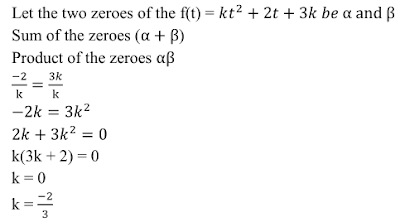9. If α and β are the zeros of the quadratic polynomial p(x) = 4x2 - 5x - 1, find the value of α2β + αβ2.

Solution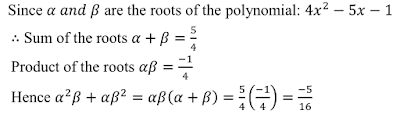10. If α and β are the zeros of the quadratic polynomial f(t) = t2 - 4t + 3 , find the value of α4β3 + α3β4.

Solution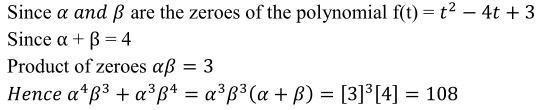11. If α and β are the zeros of the quadratic polynomial f(x) = 6x2 + x - 2 , find the value of α/β + β/α.

Solution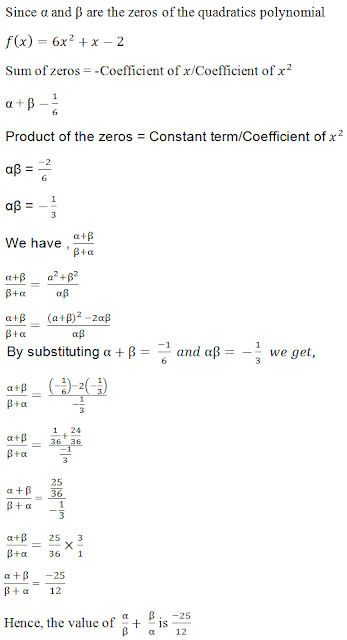12. If α and β are the zeros of the quadratic polynomial p(s) = 3s2 – 6s + 4, find the value α/β + β/α + 2 (1/α + 1/β) + 3αβ .

Solution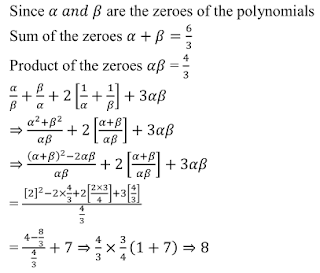13. If the squared difference of the zeros of the quadratic polynomial f(x) = x2 + px + 45 is equal to 144, find the value of p.

Solution14. If α and β are the zeros of the quadratic polynomial f(x) = x2 – px + q , prove that α22 + β2/ α2 = p4/q2 – 4p2/q + 2

Solution15. If α and β are the zeros of the quadratic polynomial f(x) = x2 – p(x+1) – c, show that (α + 1) (β+1) = 1 – c .

Solution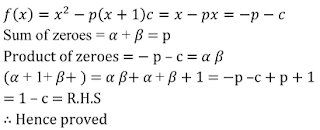16. If α and β are the zeros of a quadratic polynomial such that α + β = 24 and α − β = 8, find a quadratic polynomial have α and β as its zeros.

Solution17. If α and β are the zeros of the quadratic polynomial f(x) = x2 − 1, find a quadratic polynomial whose zeroes are 2 α/β and 2 β/α

Solution18. If α and β are the zeros of the quadratic polynomial f(x) = x2 − 3x − 2, find a quadratic polynomial whose zeroes are 1/2α + β + 1/2β + α .

Solution19. If α and β are the zeros of the polynomial f(x) = x2 + px + q , form a polynomial whose zeroes are (α+ β)2 and (α – β)2

Solution20. If α and β are the zeros of the quadratic polynomial f(x) = x2 – 2x + 3, find a polynomial whose roots are (i) α+2, β+2 (ii) α–1/α+1, β–1/ β+1

Solution21. If α and β are the zeros of the quadratic polynomial f(x) = ax2 + bx + c, the evaluate :-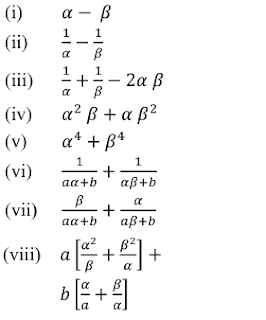Solution